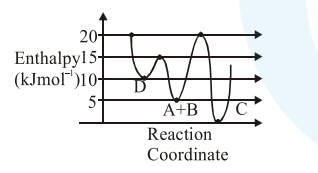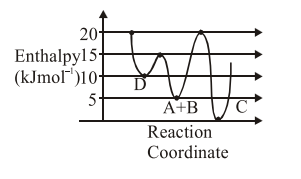# Consider the given plot of enthalpy of

Question:

Consider the given plot of enthalpy of the following reaction between $\mathrm{A}$ and $\mathrm{B}$.

$\mathrm{A}+\mathrm{B} \rightarrow \mathrm{C}+\mathrm{D}$

Identify the incorrect statement.1. $\mathrm{C}$ is the thermodynamically stable product.

2. Formation of $\mathrm{A}$ and $\mathrm{B}$ from $\mathrm{C}$ has highest enthalpy of activation.

3. D is kinetically stable product.

4. Activation enthalpy to form $\mathrm{C}$ is $5 \mathrm{~kJ} \mathrm{~mol}^{-1}$ less than that to form D.

Correct Option: , 4

Solution:

$\mathrm{A}+\mathrm{B} \rightarrow \mathrm{C}+\mathrm{D}$Activation enthalpy for $\mathrm{C}=20-5=15 \mathrm{~kJ} / \mathrm{mol}$

Activation enthalpy for $\mathrm{D}=15-5=10 \mathrm{~kJ} / \mathrm{mol}$